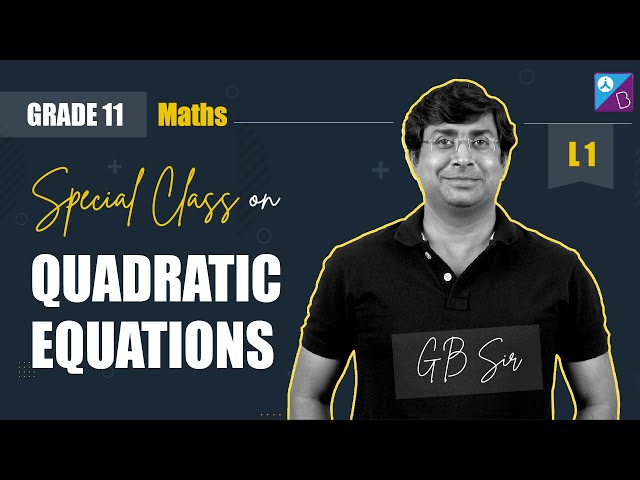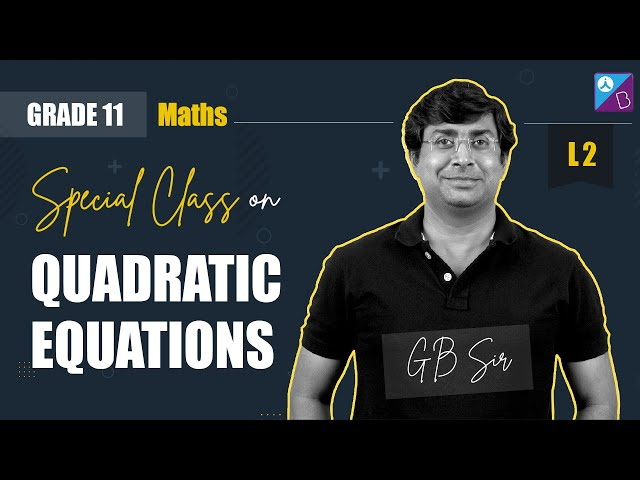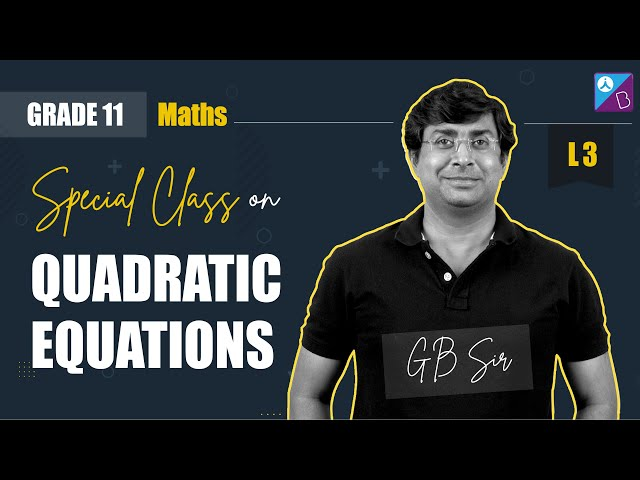Quadratic Equations Class 11 Notes are available here for students. The notes are very helpful to have a quick revision before exams. Class 11 Maths Chapter 5 quadratic equations include a quadratic formula to find the solution of the given equation.

Consider the quadratic equation: px+qx + r = 0 with real coefficients p, q, r and p≠0. Now, let us assume that the discriminant d < 0 i.e., b2-4ac< 0.

The solution of above quadratic equation will be in the form of complex numbers given by:

 $$\begin{array}{l}x=\frac{-b\pm \sqrt{b^{2}-4ac}}{2a}=\frac{-b\pm i\sqrt{4ac-b^{2}}}{2a}\end{array}$$

Important Notes:

1. A polynomial equation has at least one root
2. A polynomial equation of degree n has n roots
3. The values of a variable, that satisfy the given equation are called roots of a quadratic equation
4. The solution to quadratic equations can also be calculated using the factorisation method
5. If α and β are the roots of a quadratic equation, then the equation is x2 – (α + β) x + αβ = 0
6. The nature of roots depends on the discriminant (D) of the quadratic equation
• If D > 0, roots are real and distinct (unequal)
• If D = 0, roots are real and equal (coincident)
• If D < 0, roots are imaginary and unequal

Find solved questions based on quadratic equations using formula.

## Video Lessons

### Quadratic Equations Special Class 1### Quadratic Equations Special Class 2### Quadratic Equations Special Class 3### Rapid Revision## Quadratic Equations Class 11 Examples

$$\begin{array}{l}\text{1. Find the roots of equation } x^{2}+2=0\end{array}$$

Solution:

$$\begin{array}{l}\text{Given, }x^{2}+2=0\end{array}$$
$$\begin{array}{l}i.e.,~ x^{2} = -2 ~ or ~x = \pm \sqrt{2}i\end{array}$$
$$\begin{array}{l}\text{2. Solve }\sqrt{5}x^{2}+x+\sqrt{5}=0\end{array}$$

Solution:

$$\begin{array}{l}\text{Given }\sqrt{5}x^{2}+x+\sqrt{5}=0\end{array}$$
$$\begin{array}{l}\text{Therefore, discriminant D = }b^{2}-4ac=1-4(\sqrt{5}\times \sqrt{5})=-19\end{array}$$
$$\begin{array}{l}\text{Therefore, the solution of given quadratic equation = }\frac{-1\pm \sqrt{-19}}{2\sqrt{5}}=\frac{-1\pm 19i}{2\sqrt{5}}\end{array}$$
$$\begin{array}{l}\text{3. Solve }x^{2}+x+1=0\end{array}$$

Solution:

$$\begin{array}{l}\text{Given, }x^{2}+x+1=0\end{array}$$
$$\begin{array}{l}\text{Therefore, discriminant D = }b^{2}-4ac=1-4=-3\end{array}$$
$$\begin{array}{l}\text{Therefore, the solution of given quadratic equation = }\frac{-1\pm \sqrt{-3}}{2}=\frac{-1\pm 3i}{2}\end{array}$$